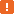# aqDateTime.Time Method### Description

Use the `aqDateTime.Time` method to get the current time. The resulting Date/Time value contains only the time portion.

To get both the current date and time, use the `Now` method. To get just the current date without time, use the `Today` method.

### Declaration

aqDateTime.Time()

 Result Date/Time

### Applies To

The method is applied to the following object:

### Result Value

A Date/Time value that contains the current time.In JavaScript, JScript, Python or DelphiScript, if you try to output the date and time returned by the `Time` method as a string, conversion takes place and the date and time are output in the native format for the selected language (`day/month/date/time/year` in JavaScript, JScript and DelphiScript, `year/month/day/time` in Python). For example, this happens if you use the following code to post the current time to the test log:

JavaScript, JScript

function Test()
{
Log.Message("Current time: " + aqDateTime.Time());
}

Python

``````def Test():
Log.Message("Current time: " + str(aqDateTime.Time()))``````

VBScript

Sub Test
Log.Message("Current time: " & aqDateTime.Time)
End Sub

DelphiScript

function Test;
begin
Log.Message('Current time: ' + aqDateTime.Time);
end;

C++Script, C#Script

procedure Test()
{
Log["Message"]("Current time: " + aqDateTime["Time"]());
}

To avoid such a conversion, you need to explicitly specify the format in which time should be displayed. For example, you can use the following code:

JavaScript, JScript

function Test()
{
Log.Message("Current time: " + aqConvert.DateTimeToFormatStr(aqDateTime.Time(), "%H:%M:%S"));
}

Python

``````def Test2():
Log.Message("Current time: " + aqConvert.DateTimeToFormatStr(aqDateTime.Time(), "%H:%M:%S"))``````

VBScript

Sub Test
Log.Message("Current time: " & aqConvert.DateTimeToFormatStr(aqDateTime.Time, "%H:%M:%S"))
End Sub

DelphiScript

procedure Test;
begin
Log.Message('Current time :' + aqConvert.DateTimeToFormatStr(aqDateTime.Time, '%H:%M:%S'));
end;

C++Script, C#Script

function Test()
{
Log["Message"]("Current time: " + aqConvert["DateTimeToFormatStr"](aqDateTime["Time"](), "%H:%M:%S"));
}

### Example

The code below demonstrates how you can compare different date and time values.

JavaScript, JScript

function ComparingDates()
{
var currentDateAndTime = aqDateTime.Now();
var currentTime = aqDateTime.Time();
// The default date for currentTime is set to 12/30/1899
var currentDate = aqDateTime.Today();
// The default time for currentDate is set to 00:00:00

Log.Message(currentDateAndTime);
Log.Message(currentTime);
Log.Message(currentDate);

Log.Message(aqDateTime.Compare(currentDateAndTime, currentTime));
// 1, because currentDateAndTime is later then currentTime

Log.Message(aqDateTime.Compare(currentDateAndTime, currentDate));
// 1, because currentDateAndTime is later then currentDate

Log.Message(aqDateTime.Compare(currentTime, currentDate));
// -1, because currentTime is earlier then currentDate
}

Python

``````def ComparingDates():
currentDateAndTime = aqDateTime.Now()
currentTime = aqDateTime.Time()
# The default date for currentTime is set to 12/30/1899
currentDate = aqDateTime.Today()
# The default time for currentDate is set to 00:00:00
Log.Message(currentDateAndTime)
Log.Message(currentTime)
Log.Message(currentDate)
Log.Message(aqDateTime.Compare(currentDateAndTime, currentTime))
# 1, because currentDateAndTime is later then currentTime
Log.Message(aqDateTime.Compare(currentDateAndTime, currentDate));
# 1, because currentDateAndTime is later then currentDate
Log.Message(aqDateTime.Compare(currentTime, currentDate));
# -1, because currentTime is earlier then currentDate``````

VBScript

Sub ComparingDates
Dim currentDateAndTime, currentTime, currentDate

currentDateAndTime = aqDateTime.Now
currentTime = aqDateTime.Time
' The default date for currentTime is set to 12/30/1899
currentDate = aqDateTime.Today
' The default time for currentDate is set to 00:00:00

Log.Message(currentDateAndTime)
Log.Message(currentTime)
Log.Message(currentDate)

Log.Message(aqDateTime.Compare(currentDateAndTime, currentTime))
' 1, because currentDateAndTime is later then currentTime

Log.Message(aqDateTime.Compare(currentDateAndTime, currentDate))
' 1, because currentDateAndTime is later then currentDate

Log.Message(aqDateTime.Compare(currentTime, currentDate))
' -1, because currentTime is earlier then currentDate
End Sub

DelphiScript

procedure ComparingDates;
var currentDateAndTime, currentTime, currentDate;
begin
currentDateAndTime := aqDateTime.Now;
currentTime := aqDateTime.Time;
// The default date for currentTime is set to 12/30/1899
currentDate := aqDateTime.Today;
// The default time for currentDate is set to 00:00:00

Log.Message(currentDateAndTime);
Log.Message(currentTime);
Log.Message(currentDate);

Log.Message(aqDateTime.Compare(currentDateAndTime, currentTime));
// 1, because currentDateAndTime is later then currentTime

Log.Message(aqDateTime.Compare(currentDateAndTime, currentDate));
// 1, because currentDateAndTime is later then currentDate

Log.Message(aqDateTime.Compare(currentTime, currentDate));
// -1, because currentTime is earlier then currentDate
end;

C++Script, C#Script

function ComparingDates()
{
var currentDateAndTime = aqDateTime["Now"]();
var currentTime = aqDateTime["Time"]();
// The default date for currentTime is set to 12/30/1899
var currentDate = aqDateTime["Today"]();
// The default time for currentDate is set to 00:00:00

Log["Message"](currentDateAndTime);
Log["Message"](currentTime);
Log["Message"](currentDate);

Log["Message"](aqDateTime["Compare"](currentDateAndTime, currentTime));
// 1, because currentDateAndTime is later then currentTime

Log["Message"](aqDateTime["Compare"](currentDateAndTime, currentDate));
// 1, because currentDateAndTime is later then currentDate

Log["Message"](aqDateTime["Compare"](currentTime, currentDate));
// -1, because currentTime is earlier then currentDate
}